# The true nature of the golden ratio

### 1, 1, 2, 3, 5, 8, 13, 21, 34…

The most discerning readers will have recognized the beginning of the famous Fibonacci sequence, in which each new term is obtained as the sum of the previous two, known for its connection with the mythical golden ratio

#####The exact value of the golden ratio.

At the beginning of the thirteenthe century, when Leonardo Fibonacci introduced this sequence in his treatise Liber Abaci to put in a very simplified manner the evolution of a group of immortal rabbits, he had no idea of ​​the importance it would acquire in mathematics, so much so that a scientific journal would be devoted entirely to it several centuries later (The Fibonacci Quarterly, created in 1963).

He also might not have realized that the terms for this sequence are frequently found in nature, not in the study of rabbit demographics…but in botany!

On the cones of pines, pineapples, or flowers of the sunflower family, we notice patterns in the form of spirals, which are organized into two intersecting networks. If curiosity leads us to calculate the spirals of these networks, we often get two consecutive numbers of the Fibonacci sequence. For example, a pine cone has 8 spirals in one direction and 13 spirals in the other. In the core of the chrysanthemum or star flower, the small flowers arranged on the head (florets) form two families of 13 and 21 spirals, or even 21 and 34.

In large flowers such as sunflowers, we find pairs (34.55) or (55.89), and perhaps more.

#####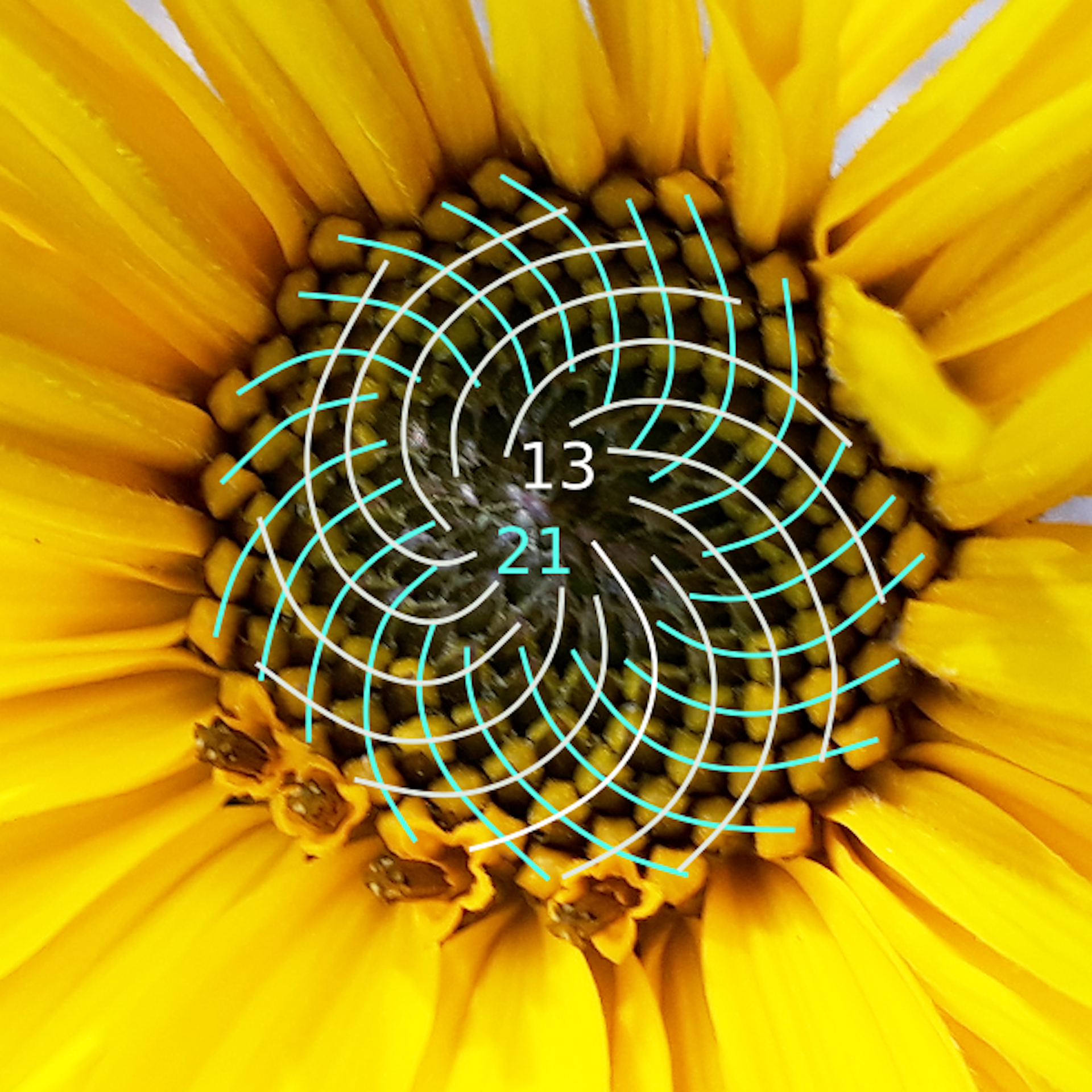A variety of chrysanthemums.

It seems hard to believe a simple coincidence: the regularity of this phenomenon seems to reveal rather the application of mathematical principles to the complex mechanisms of plant growth.

These are the works of botany, the science that studies the (geometric) arrangements of the leaves, flowers, or petals of plants, which shed light on these principles from the nineteenth century.

Then mathematicians and computer scientists looked at the question, and worked on models to describe the mechanisms of plant formation in a simplified way.

### sunflower pattern

We have produced an online application that demonstrates the operation of one of these models, based on the work of Helmut Vogel.

The same type of model was used in this excellent video (in English) from Numberphile, which also drew us from the following explanations.

This model is based on a general principle: plant organs such as leaves, petals or florets grow here one by one, each time forming a constant angle with the previous organ. This angle is calleddivergence angleand is usually expressed as a ratio of the complete turnover.

Thus, a spacing angle of 1/6 means that one rotates one-sixth of a turn around the center of the head to place the next flower. If the position of the new flower after this rotation overlaps with the already existing flower, then the distance from the center of the head increases so that it deviates sufficiently from the old flower.

#####Build start to 1/6 spacing angle.

In this model, the process ends when one reaches the edge of a disk of previously fixed size. What number do we get next? Its shape strongly depends on the value of the angle of divergence. Let’s first see the result of simple values.

### branches in flowers

When you turn a quarter turn (first picture), 4 straight branches form, which can be easily explained: if you repeat this process 4 times, it will go back in the direction of the start. Similarly, one-sixth of a turn gives 6 branches (second image). When the angle of divergence is equal to 11/23 (third picture), then 23 repetitions of the rotation correspond to 11 complete revolutions, and therefore also leads to the starting direction: we then get 23 straight branches.

From the point of view of the plant, these angles of difference are not really interesting, because a large part of the available space in the head is not used. This problem appears every time we choose a logical divergence angle, ie one written as the quotient of two integers. So it’s tempting to test what happens when the angle of difference doesn’t make sense. Then we say it’s irrational: we can’t write it as the quotient of two integers.

The first example of an irrational number that comes to mind is the famous number π, which occurs in calculating the circumference of a circle and in many other mathematical formulas. The following figure shows the result obtained if we choose a spacing angle equal to 1 / π.

### The number π is very logical!

It’s curious that while π (and thus also 1/π) is not a rational number, the number is of the same type obtained for a rational as 11/23, here with 22 branches. However these branches are slightly curved here.

A closer examination of the given figure of 1/ will allow us to better understand the expected characteristics of the angle of divergence of the plant. Let’s start by noticing what happens towards the center: the process begins with the formation of 3 curved strands.

We can easily explain the existence of these three branches: the decimal writing of π starts with 3.14159 … So in the first approximation, π is close to 3. So the number 1 / π is close to 1/3. However, 1/3 divergence angle will give a shape with 3 straight branches, so what happens towards the center is an approximation of this behaviour.

These three branches are curved here, because 1/ doesn’t exactly equal 1/3. Then, when new flowers form, the distance to the center increases, and approaching the spacing angle by 1/3 becomes very coarse. Then the three central branches end up disappearing.

How then do we explain the formation of 22 new branches? Another simple approximation of the number, much more accurate than 3, is obtained by the fraction 22/7≃3.142857.

The angle of divergence is thus very well approximated by the fraction 7/22. If it is 7/22, then we will notice 22 straight branches. Since the approximation is pretty good but not accurate, we’ve got these 22 branches slightly curved.

Even if 1/ is not a rational number, it is far from optimal, since a lot of space is lost on the flower head: on the same surface we can put several florets that would give more seeds! The missing surface comes from the fact that the spacing angle 1/ is well approximated by rational numbers. In order for the florets to be arranged in a very efficient way on the head of the flower, it will be necessary to use a spacing angle that is poorly approximated as possible by rational numbers.

### The golden ratio comes into effect

The Diophantine approximation problem (how to round any number with rational numbers) is related to the theorem continuous fractures, which consists of representing any number in the form of a fraction, possibly infinite when the number is irrational. For example, the number 1 / can be written as

#####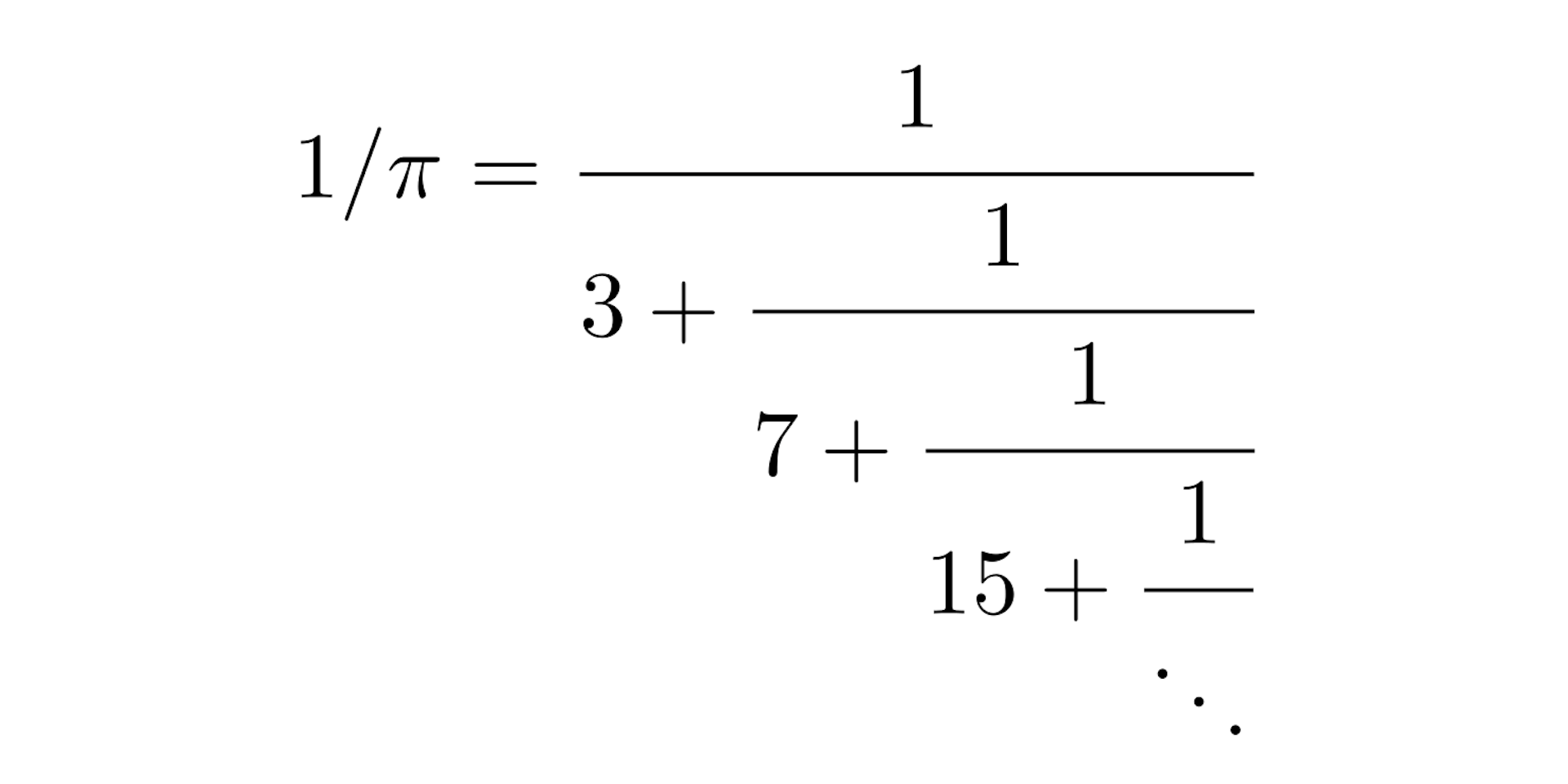Write the inverse of π as a function fraction.

Where the fracture continues indefinitely.

By subtracting this infinitesimal fraction, we get the best approximations of an irrational number given by rational numbers: the so-called drop. For example, stopping the fraction at 7, we get the reduced 7/22 as a good approximation of 1/π.

#####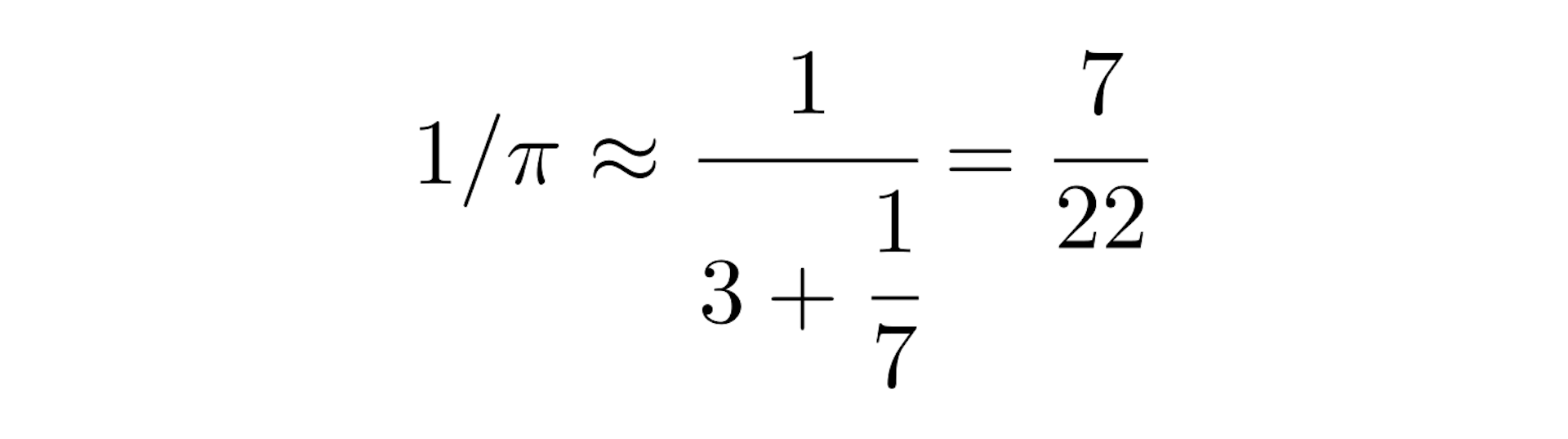Rounding off the inverse of π by one of its reducers.

However, there is a number in which these reductions remain as far apart as possible: the number whose continuous fraction expansion contains only “1s”. It’s written

#####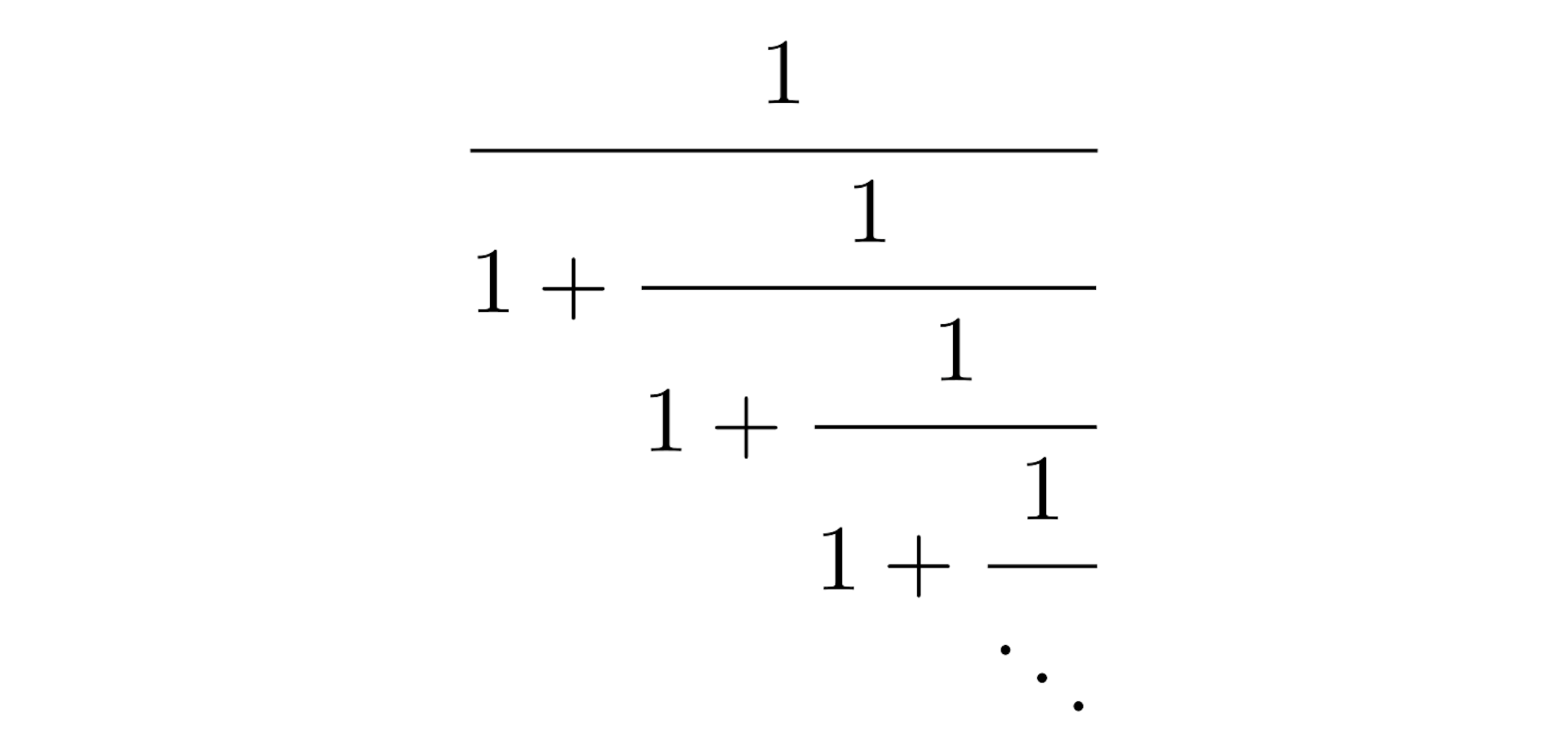Continuous fraction to reverse the golden ratio.

But this number is exactly the inverse of the golden ratio:

#####The inverse of the golden ratio.

This theorem thus leads us to the optimal choice of the divergence angle, which is the worst angle approximated by rational numbers: it is the angle whose ratio with the full revolution is the inverse of the golden ratio, which is calledgolden angle. Let’s see the given figure in this case.

#####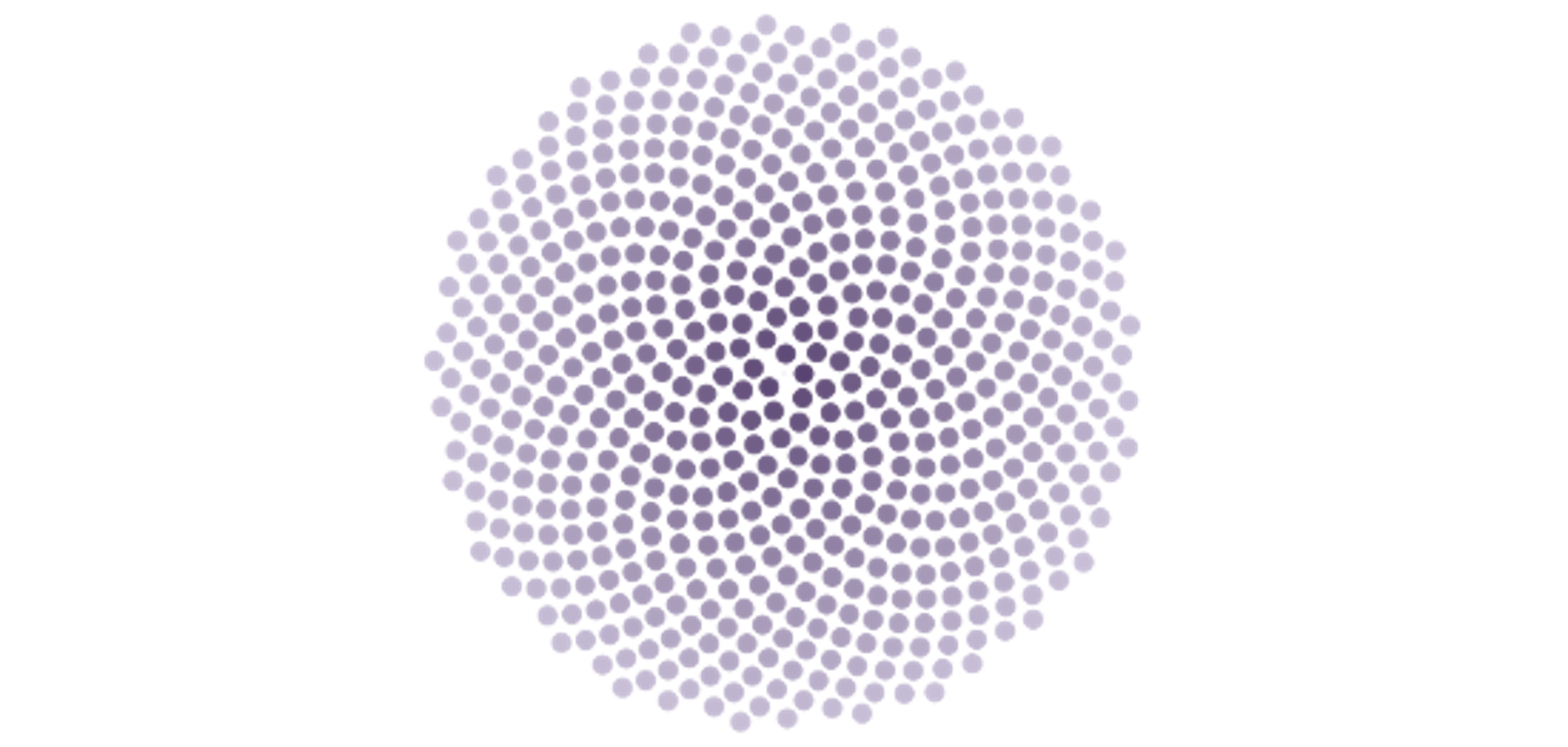Simulation when the angle of divergence is equal to the golden angle 1/.

In fact, in the golden corner we see a better use of the available space: the florets are arranged regularly without leaving much empty space on the head of the flower. There is also a real resemblance to the arrangement observed on real sunflowers.

### Fibonacci in all of this?

The spirals in the form of the golden angle correspond to the “branches” observed in cases of rational (roughly) divergence: each network of spirals is associated with a rational approximation of the golden angle to one of its reduced angles. As in the cases 1/6, 11/23 or 1/, the number of spirals (or branches) is given by the denominator of the fraction specified by the reducer. However, golden angle cuts have consecutive denominators… Fibonacci numbers!

Thus the presence of Fibonacci numbers in the spirals of plants is the signature of the wonderful golden number.

The particular difficulty of approaching the fractions led to his selection by the natural development of many plants. Remarkably, mathematical theories which are considered axiomatic very far from botany shed appropriate light in this field.